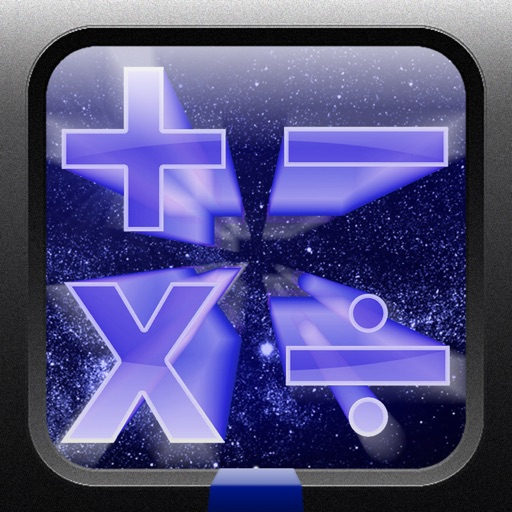Mathematics - addition , subtraction , multiplication , division# Mathematics - addition , subtraction , multiplication , division

\$0.99
0

0 Ratings

Update Date

2013-11-21

Size

2.4 MB

Mathematics - addition , subtraction , multiplication , division Description
Mathematics is a learning app for everyone.
Train or learn your basic math knowledge
- Choose individually addition, subtraction, multiplication, or division, some of them or all together,
- Set minimum and maximum numbers, or select the number of multiplication / division
- The tasks appear in random order
- Detailed evaluation after each training or test
- Statistics
- Tests with or without time limits (in three stages)

options:
- Subtraction
- Multiplication
- Division  training

Will you calculate against time?
*Timer:
- 8 Sec.
- 16 Sec.
- 20 Sec. or Off

learn math with fun!!!
Mathematics - addition , subtraction , multiplication , division 1.0.2 Update
2013-11-21 Version History
- Bugs fixes
More